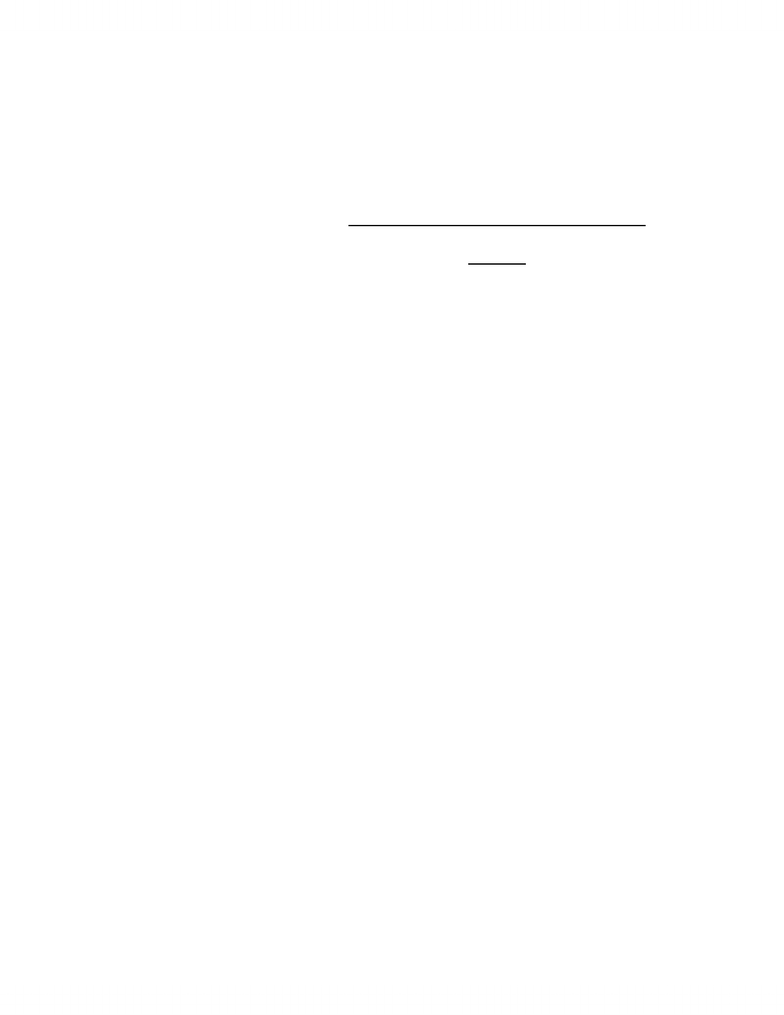# Economics 1021A/B Chapter Notes - Chapter 4: Negative Number, Longrun, Inferior Good

15 views5 pages
School
DepartmentChapter 4 Notes
Price Elasticity of Demand
the price elasticity of demand is a units-free measure of the responsiveness of the
quantity demanded of a good to a change in its price, ceteris parabus
  

  

in this calculation, the average price and average quantity are used
when the price of a good rises, the quantity demanded decreases
o because a positive change in price brings a negative change in the quantity
demanded, the price elasticity of demand is a negative number
o we take the absolute value of this
Elastic and Inelastic Demand
if the quantity demanded remains constant when the price changes, then the price
elasticity of demand is zero, and the good is said to have a perfectly inelastic
demand
o e/x insulin, people will buy the same amount regardless of price
if the percentage change in the quantity demanded equals the percentage change in
the price, then the price elasticity equals 1, and the good is said to have a unit elastic
demand
if the price elasticity of demand of a good is between zero and 1, it is said to have an
inelastic demand
if the quantity demanded changes by an infinitely large percentage in response to a
tiny price change, then the price elasticity of demand is infinity and the good is said
to have a perfectly elastic demand
if price elasticity of demand is greater than 1, it is said to have an elastic demand
o e/x cars, furniture
Total Revenue and Elasticity
the total revenue from the sale of a good equals the price of the good multiplied by
the quantity sold
the change in total revenue depends on the elasticity of demand in the following
ways:
o if demand is elastic, a 1% price cut increases the quantity sold by more than
1% and total revenue increases
o if demand is inelastic, 1% price cut increases the quantity sold by less than 1%
and total revenue decreases
o if demand is unit elastic, a 1% price cut increases the quantity sold by 1% and
total revenue does not change
Unlock document

This preview shows pages 1-2 of the document.
Unlock all 5 pages and 3 million more documents.the total revenue test is a method of estimating the price elasticity of demand by
observing the change in total revenue that results from a change in price, ceteris
parabus
o if a price cut increases total revenue, demand is elastic
o if a price cut decreases total revenue, demand is inelastic
o if a price cut leaves total revenue unchanged, demand is unit elastic
personal expenditure
o when a price changes, the change in your expenditure on the good depends on
one’s own elasticity of demand
if YOUR demand is elastic, a 1% price cut increases the quantity you
buy by more than 1%, personal expenditure increases
if YOUR demand is inelastic, a 1% price cut increases the quantity
you buy by less than 1%, personal expenditure decreases
if YOUR demand is unit elastic, a 1% price cut increases the quantity
you buy by 1% and your expenditure on the item does not change
Factors Influencing Elasticity of Demand
closeness of substitutes
o the closer the substitutes for a good/service, the more elastic is the demand for
it
e/x few substitutes for gasoline, so demand for oil is inelastic
o a necessity has poor substitutes, meaning that they generally have inelastic
demand
o a luxury usually has many substitutes, meaning that they generally have an
elastic demand
proportion of income spend on the good
o the greater the proportion of income spent on a good, the more elastic (or less
inelastic) is the demand for it
time elapsed since price change
o the longer the time that has elapsed since a price change, the more elastic is
demand
Cross Elasticity of Demand
the cross elasticity of demand is a measure of how the responsiveness of the demand
for a good to change in the price of a substitute or complement, ceteris parabus
  

  

the cross elasticity of demand can be positive or negative
o it is positive for a substitute, negative for a complement
substitutes
o when the price of a substitute rises, the demand for your good increases
o cross elasticity is positive
Unlock document

This preview shows pages 1-2 of the document.
Unlock all 5 pages and 3 million more documents.

## Document Summary

Price elasticity of demand the price elasticity of demand is a units-free measure of the responsiveness of the quantity demanded of a good to a change in its price, ceteris parabus. Closeness of substitutes the closer the substitutes for a good/service, the more elastic is the demand for it. E/x few substitutes for gasoline, so demand for oil is inelastic: a necessity has poor substitutes, meaning that they generally have inelastic demand, a luxury usually has many substitutes, meaning that they generally have an elastic demand. Substitutes: when the price of a substitute rises, the demand for your good increases, cross elasticity is positive. Compliments: when the price of a compliment rises, the demand for your good increases, cross elasticity is negative. Factors influencing the elasticity of supply resource substitution possibilities: some goods and services can be produced only by using unique or rare productive resources. Such items have a low elasticity of supply.

# Get access

\$10 USD/m
Billed \$120 USD annually
Homework Help
Class Notes
Textbook Notes